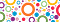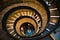# Experimental Math — Computing Units of Modular RingsImage by Gerd Altmann from Pixabay

A ring is an algebraic structure modelled after the integers and their arithmetic properties. Formally, a ring is defined as a structure consisting of a nonempty set, S, and two operations; addition(+) and multiplication(*) satisfying the following properties for all x, y, z in S:

1. x + y is also a member of S (closure under addition)
2. x + y = y + x (additive commutativity)
3. x + (y + z)= (x + y) + z (associativity with respect to addition)
4. There is an element 0, such that x + 0 = 0 + x = x for all x

# Getting Down with Fermat — A Lesson in Infinite DescentPhoto by YIFEI CHEN on Unsplash

Pierre de Fermat, a seventeenth century French lawyer and mathematician needs no introduction, he is famous for the notoriously hard conjecture, Fermat’s Last Theorem(FLT), which took over three centuries and a half to prove after a series of failed attempts by cranks and professional mathematicians alike.

It is impossible to separate a cube into two cubes, a fourth power into two fourth powers, or generally, any power above the second into two powers of the same degree”, Fermat wrote in the margin of his copy of an ancient math text. What that means in mathematical notation is that there are…

# A first look at Groups

A group is a monoid in which every element has an inverse.

Fact of life.

I always chuckle when I think of that college textbook definition of a group. Innocently waggish though very correct.

While that opening definition of a group is correct, it doesn’t immediately convey the essence of a group, especially to a math noob.

Groups are ubiquitous objects in mathematics with diverse practical and theoretical applications. We are going to be looking at the meaning of a group and how the idea develops, but first, a look at sets and binary operations.

# Sets and Binary Operations

One of the fundamental notions…## Akintunde Ayodele

Programmer. Mathematician. Thinker. Researcher at ZetaLabs, Lagos, Nigeria.